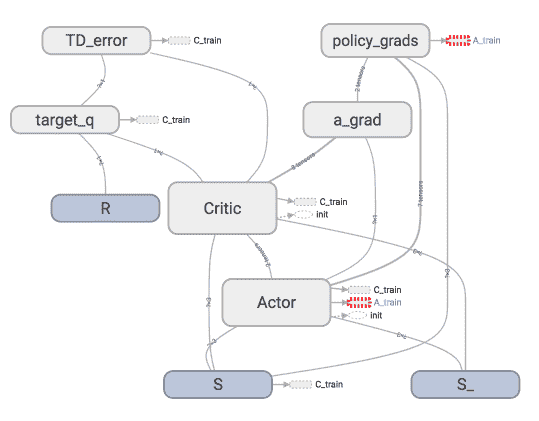# 5.1 – 为什么 Torch 是动态的

## 动态?静态?## 动态RNN

..

######################## 前面代码都一样, 下面开始不同 #########################

################ 那节内容的代码结构 (静态 time step) ##########
for step in range(60):
start, end = step * np.pi, (step 1)*np.pi   # time steps 都是一样长的
# use sin predicts cos
steps = np.linspace(start, end, 10, dtype=np.float32)
...

################ 这节内容修改代码 (动态 time step) #########
step = 0
for i in range(60):
dynamic_steps = np.random.randint(1, 4)  # 随机 time step 长度
start, end = step * np.pi, (step   dynamic_steps) * np.pi  # different time steps length
step  = dynamic_steps

# use sin predicts cos
steps = np.linspace(start, end, 10 * dynamic_steps, dtype=np.float32)

#######################  这下面又一样了 ###########################
print(len(steps))   # print how many time step feed to RNN

x_np = np.sin(steps)    # float32 for converting torch FloatTensor
y_np = np.cos(steps)
...

"""

30
30
10
30
20
30
"""